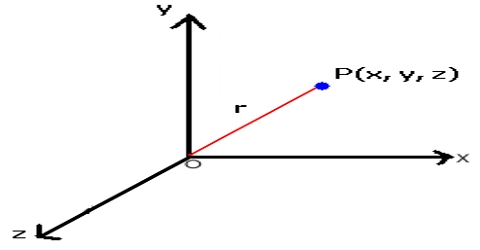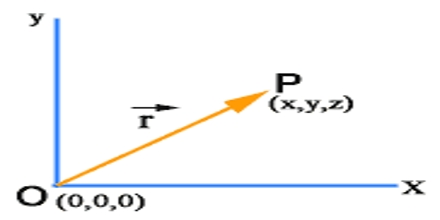# Position Vector

A vector is needed in order to know the position of a point in the reference frame. The position of the body can be determined by the magnitude and direction of that vector. Physicists use the concept of a position vector as a graphical tool to visualize displacements. A position vector expresses the position of an object from the origin of a coordinate system.

When the position of a sector is specified with reference to origin of a reference frame, that sector is called position vector.As the point moves, the position vector will change in length or in direction or in both length and direction. Suppose X and Y are two mutually perpendicular axes and O is their origin, P is any Point. Here OP vector indicates the position of point P with respect to point O. So, OP is a position vector [Figure].